## Orthogonal Matrix

Any Rotation can be given as a composition of rotations about three axes (Euler's Rotation Theorem), and thus can be represented by aMatrix operating on a Vector,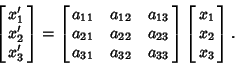(1)

We wish to place conditions on this matrix so that it is consistent with an Orthogonal Transformation (basically, a Rotation or Rotoinversion).

In a Rotation, a Vector must keep its original length, so it must be true that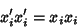(2)

for, 2, 3, where Einstein Summation is being used. Therefore, from the transformation equation,(3)

This can be rearranged to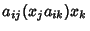(4)

In order for this to hold, it must be true that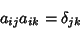(5)

for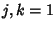, 2, 3, where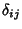is the Kronecker Delta. This is known as the Orthogonality Condition, and it guarantees that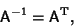(6)

and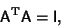(7)

where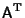is the Matrix Transpose and I is the Identity Matrix. Equation (7) is the identity which gives the orthogonal matrix its name. Orthogonal matrices have special properties which allow them to be manipulated and identified with particular ease.

Letand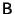be two orthogonal matrices. By the Orthogonality Condition, they satisfy(8)

and(9)

whereis the Kronecker Delta. Now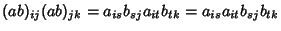(10)

so the product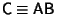of two orthogonal matrices is also orthogonal.

The Eigenvalues of an orthogonal matrix must satisfy one of the following:

1. All Eigenvalues are 1.

2. One Eigenvalue is 1 and the other two are.

3. One Eigenvalue is 1 and the other two are Complex Conjugates of the form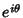and.

An orthogonal Matrixis classified as proper (corresponding to pure Rotation) if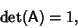(11)

whereis the Determinant of A, or improper (corresponding to inversion with possible rotation; Rotoinversion) if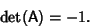(12)

See also Euler's Rotation Theorem, Orthogonal Transformation, Orthogonality Condition, Rotation, Rotation Matrix, Rotoinversion

References

Arfken, G. Orthogonal Matrices.'' Mathematical Methods for Physicists, 3rd ed. Orlando, FL: Academic Press, pp. 191-205, 1985.

Goldstein, H. Orthogonal Transformations.'' §4-2 in Classical Mechanics, 2nd ed. Reading, MA: Addison-Wesley, 132-137, 1980.